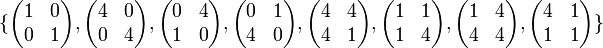# 2-Sylow subgroup of special linear group:SL(2,5)

(diff) ← Older revision | Latest revision (diff) | Newer revision → (diff)$G$ is the special linear group:SL(2,5), i.e., the special linear group of degree two over field:F5. In other words, it is the group of invertible$2 \times 2$ matrices of determinant 1 over the field with three elements. The field has elements 0,1,2,3,4 with$4 = -1$.$H$ is the subgroup:$\{ \begin{pmatrix} 1 & 0 \\ 0 & 1 \\\end{pmatrix}, \begin{pmatrix} 4 & 0 \\ 0 & 4 \\\end{pmatrix}, \begin{pmatrix} 0 & 4 \\ 1 & 0 \\\end{pmatrix}, \begin{pmatrix} 0 & 1 \\ 4 & 0 \\\end{pmatrix}, \begin{pmatrix} 4 & 4 \\ 4 & 1 \\\end{pmatrix}, \begin{pmatrix} 1 & 1 \\ 1 & 4 \\\end{pmatrix}, \begin{pmatrix} 1 & 4 \\ 4 & 4 \\\end{pmatrix}, \begin{pmatrix} 4 & 1 \\ 1 & 1 \\\end{pmatrix} \}$